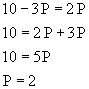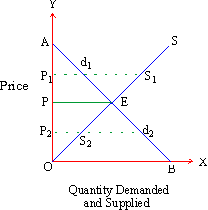Home MonkeyNotes Printable Notes Digital Library Study Guides Study Smart Parents Tips College Planning Test Prep Fun Zone Help / FAQ How to Cite New Title Request

 2.5 The Concept of Equilibrium Both demand and supply functions independently serve important functions. However, it is important to bring them together in an attempt to establish equilibrium. The concept of equilibrium, though analytical in nature, is quite simple in practice. It can be defined as a point of equality or agreement between buyers and sellers. Since both demand and supply quantities are shown in the scheduled forms these indicate mutual willingness of consumers and producers to purchase or sell respectively, varying quantities at varying prices. The schedules do not yet explain actual market price at which deals take place. This can be possible only when the quantities demanded and supplied are exactly equal at some uniform price. So long as this has not been achieved, some buyers or sellers are yet dissatisfied and may attempt to raise or lower the price. In this sense equilibrium is a point of complete satisfaction of the given behavior of buying and selling and hence an act of fulfillment of a given economic activity. Lets present and illustrate the establishment of equilibrium with the help of demand and supply functions in our earlier examples (in the sections given above). We begin with two equations: qd = 10 - 3P and qs = 2P By definition, demand and supply must be equal (qd = qs) for the condition of equilibrium. qd = 10 - 3P = qs = 2 P or 10 - 3P = 2P On solving this we find equilibrium price:On substituting the value of price in demand and supply function we get, qd = 10 - 3P qd = 10 - 3 (2) = 10 - 6 = 4 qs = 2P qs = 2 (2) = 4 Hence equilibrium price is 2, at which both quantity demanded and supplied are equal to 4. The algebraic proof (Figure 7) of the equilibrium can also be presented geometrically.Figure 9 In the figure, AB and OS are the demand and supply curves respectively. The two curves intersect at point E which is an equilibrium point at which price P = 2 and quantity demanded and supplied are both equal (q = 4). At any other price higher than P such as P1, the quantity supplied S1 exceeds the quantity demanded d1 (S1 > d1) and hence at this stage, some sellers remain dissatisfied. On the other hand at any lower price such as P2, quantity demanded d2 exceeds quantity supplied S1 (d2 > S1) and this time some buyers remain dissatisfied. Therefore only at the point of intersection between demand and supply curves can equilibrium be attained. In other words, equilibrium price represents that price at which buyers are willing to buy the good and sellers are willing to sell it. This is the point of satisfaction for both the groups.   ********** Index 2. 1 Fundamental Concepts 2. 2 Demand Schedule, Function and Law 2. 3 Supply Schedule, Function and Law 2. 4 Elasticity of Demand and Supply 2. 5 The Concept of Equilibrium Chapter 3
 Search: All Products Books Popular Music Classical Music Video DVD Toys & Games Electronics Software Tools & Hardware Outdoor Living Kitchen & Housewares Camera & Photo Cell Phones Keywords: